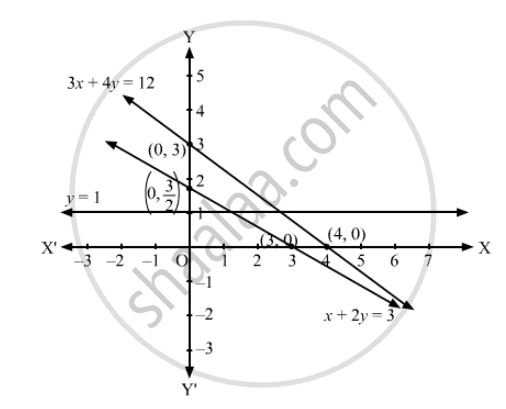Department of Pre-University Education, KarnatakaPUC Karnataka Science Class 11
Advertisement Remove all ads

# How that the Solution Set of the Following Linear Inequations is Empty Set: X + 2y ≤ 3, 3x + 4y ≥ 12, Y ≥ 1, X ≥ 0, Y ≥ 0 - Mathematics

Show that the solution set of the following linear inequations is empty set:

x + 2y ≤ 3, 3x + 4y ≥ 12, y ≥ 1, ≥ 0, y ≥ 0

Advertisement Remove all ads

#### Solution

Converting the inequations to equations, we obtain:
x + 2y = 3, 3x + 4y =12,  y = 1

x + 2y = 3:  This line meets the x-axis at (3, 0) and y-axis at (0, 3/2). Draw a thick line joining these points.
We see that the origin (0, 0) satisfies the inequation x + 2y ≤ 3. So, the portion containing the origin represents the solution set of the inequation x + 2y ≤ 3

3x + 4y =12:  This line meets the x-axis at (4, 0) and y-axis at (0, 3). Draw a thick line joining these points.
We see that the origin (0, 0) does not satisfy the inequation 3x + 4y$\geq$12 So, the portion not containing the origin represents the solution set of the inequation 3x + 4y$\geq$12y = 1:  This line is parallel to x-axis at a distance of 1 unit from it.
We see that the origin (0, 0) does not satisfy the inequation y $\geq$1 So, the portion not containing the origin represents the solution set of the inequation y $\geq$1
Clearly, x ≥ 0, y ≥ 0 represents the first quadrant.
We see in the figure that there is no common region in all the lines. Hence, the solution set to the given set of inequations is empty.Is there an error in this question or solution?
Advertisement Remove all ads

#### APPEARS IN

RD Sharma Class 11 Mathematics Textbook
Chapter 15 Linear Inequations
Exercise 15.6 | Q 2.2 | Page 30
Advertisement Remove all ads

#### Video TutorialsVIEW ALL 

Advertisement Remove all ads
Share
Notifications

View all notifications

Forgot password?